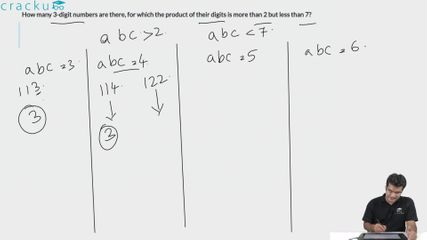Question 53

# How many 3-digit numbers are there, for which the product of their digits is more than 2 but less than 7?

Solution

Let the number be 'abc'. Then, $$2<a\times\ b\times\ c<7$$. The product can be 3,4,5,6.

We can obtain each of these as products with the combination 1,1, x where x = 3,4,5,6. Each number can be arranged in 3 ways, and we have 4 such numbers: hence, a total of 12 numbers fulfilling the criteria.

We can factories 4 as 2*2 and the combination 2,2,1 can be used to form 3 more distinct numbers.

We can factorize 6 as 2*3 and the combination 1,2,3 can be used to form 6 additional distinct numbers.

Thus a total of 12 + 3 + 6 = 21 such numbers can be formed.

### View Video Solution